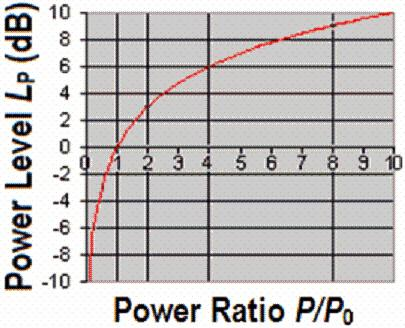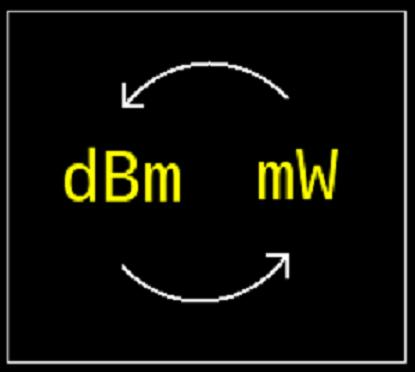# Difference Between dB and dBm in Fiber Optics

Fiber optics is a way of communication that involves transmitting information in the form of light from one place to another.

While studying about fiber optics and trying to understand its different applications, one often comes across the terms dB and dBm.

The terms are bound to sound confusing to someone who is not familiar with what they mean and denote, which pretty much makes it impossible to differentiate between them. Therefore, one must start off by learning what dB and dBm stand for.

While both dB, which stands for decibel, and dBm, which stand for dB relative to a mill-watt, are related to fiber optics, they both represent concepts that are pretty different.

A dB is a unit for the strength of a signal, which basically expresses a ratio of two power levels. A dBm, on the other hand, is an abbreviation for power ratio in dB and expresses the absolute value of power.

Unlike dB, which is merely a ratio between two powers, dBm measures power level in reference to one milli-watt.

To calculate the ratio between two power levels in dB, one needs to use the formula “log(P1/P2),” whereas the formula to used to calculate dBm is “10log(P/1mW).”

When the comparison between two signal strengths needs to be kept simple,  dB is preferred over dBm, as it makes it possible to contrast between two different power levels with a relatively simple, two-to-three digit number rather than a complex nine-to-ten digit one.

A dBm is an absolute unit as it is referenced to the watt. The dB, on the other hand, simply quantifies the ratio between differing power levels and is therefore a dimensionless unit.

Despite being a dimensionless unit, dB is part of the International System of Units and is acceptable, while the dBm is not. The latter is discouraged from being used in systems and documents that stick to SI units.

### Instructions

• 1

dB

dB stands for decibels, which is a short-hand way to express the ratio between two power levels and values such as the signal-to-noise ratio.

- Image Courtesy: sengpielaudio.com• 2

dBm

dBm stands for decibels to milli-watt and basically denotes the power ratio in dB of two power levels in reference to one milli-watt. It is typically used to measure absolute power in fiber optics network, radio and microwave.

- Image Courtesy: ekalk.eu•